# Chapter 5 Basic data analysis with Excel

In chapter 2, we learned how to obtain and clean a simple data set. The next step is to learn some tools for analyzing it. We will start with univariate statistics, i.e., statistics that describe a single variable. Later in the term we will learn some multivariate statistics that describe the relationship between two or more variables.

Chapter goals

In this chapter we will learn how to:

• Calculate and interpret the main univariate summary statistics in Excel
• Construct and interpret frequency tables in Excel
• Construct and interpret bar graphs, histograms and time series graphs in Excel

In the next chapter, we will use the tools of probability and random variables to understand these statistics more deeply.

## 5.1 Exploratory data analysis

Our emphasis in this chapter, and in much of this course will be on performing exploratory data analysis. Exploratory data analysis is the first step in any data analysis project: we use simple statistics and graphs to identify and understand patterns in the data. Our knowledge of these patterns can then inform our subsequent formal or model-based analysis.

The audience for exploratory data analysis is the analyst themselves. But we will often be interested in presenting the patterns we have discovered to another audience: your teacher, your boss, or your client. So we will also discuss how to effectively present your results.

### 5.1.1 Looking at the data

The first step in any exploratory data analysis is to literally look at the data.

Historical employment data for Canada

Our analysis in this chapter will use historical employment data for Canada from January 1976 through January 2021. Download the file at https://bvkrauth.github.io/files/CanadaEmploymentHistorical.xlsx, make a working copy, and open it.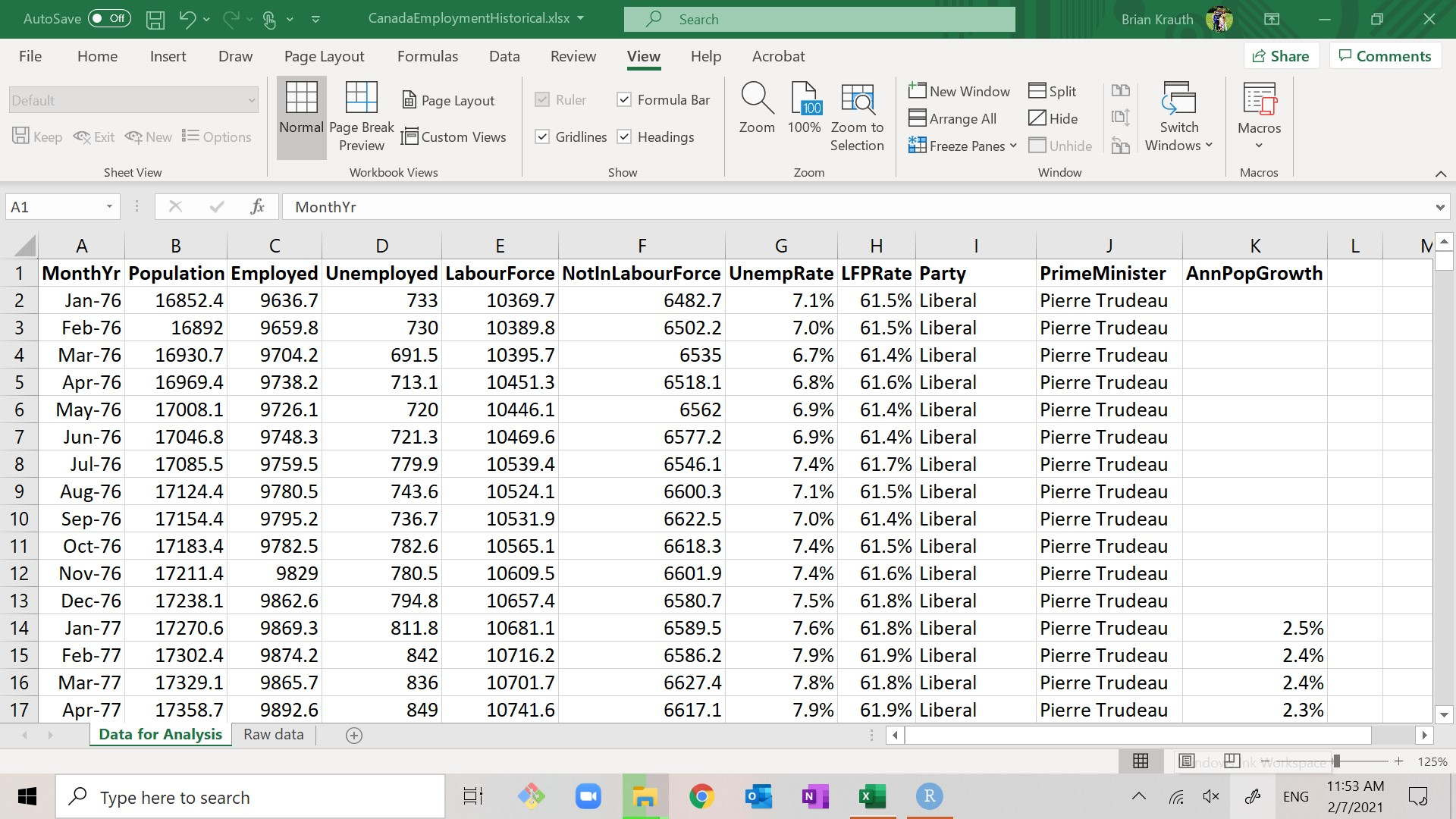Canadian employment data, 1976-2021

Our main data set for analysis is the worksheet “Data for Analysis”, which includes the following variables:

• MonthYr: the month and year of the observation.
• Population: the civilian, non-institutionalized working-age population of Canada at that time, in thousands.
• Employed: the total number employed in the population, in thousands.
• Unemployed: the total number employed in the population, in thousands.
• LabourForce: the sum of Employed and Unemployed.
• NotInLabourForce: the difference between Population and LabourForce.
• UnempRate: the percentage of the labour force that is unemployed. As before, it is calculated and stored as a decimal (ranging from 0.0 to 1.0) but displayed as a percentage (ranging from 0% to 100%).
• LFPRate: the percentage of the population that is in the labour force. As before, it is calculated and stored as a decimal (ranging from 0.0 to 1.0) but displayed as a percentage (ranging from 0% to 100%).
• Party: the political party in control of the Federal government. If the party in control changed during the month, it is listed as “Transfer”.
• PrimeMinister: the name of the Prime Minister. If the prime minister changed during the month, it is listed as “Transfer”.
• AnnPopGrowth: the rate of population growth over the previous 12 months, calculated as a proportion and displayed as a percentage. Note that this variable is blank for the first 12 months of the data set.

In addition, there is a worksheet titled “Raw data” that contains the original data as obtained from Statistics Canada. Source information is also in that worksheet.

Our historical employment data set covers more than 500 months. Other data sets are often much larger: large surveys from Statistics Canada can have hundreds of observations and hundreds of thousands of variables, and companies and governments often work with transactions-level data that includes millions of observations.

As humans, our brains are not large enough to fully understand a large dataset without some kind of simplification or “dimension reduction”: instead of looking at millions of numbers and trying to identify patterns from that, we calculate and view a relatively small number of statistics based on the data. A statistic is just a number calculated from data.

## 5.2 Univariate statistics in Excel

We will start by calculating some commonly-used univariate statistics, which are statistics that describe a single variable in isolation. In Section 5.3.1 we will construct some commonly-used univariate graphs.

Multivariate statistics describe the relationships among multiple variables, and will be coverd in Chapter 12.

### 5.2.1 Summary statistics

A table of summary statistics reports various univariate statistics for each variable in our data set. For example, our table of summary statistics might look like this:

Statistic (variable name 1) (variable name 2) (etc.)
Count (count of valid observations)
Average (average)
StdDev (standard deviation)
Min (minimum value observed)
10th percentile
Median
90th percentile
Max (maximum value observed)

You saw many of these words - standard deviation, percentile, median, etc. - in Chapter 4, but I need to be clear on something: even though the names are the same, the concepts are not exactly the same.

1. The count or sample size is the number of observations with valid (numeric) values for the variable.
2. The sample average is a measure of central tendency in data, and is calculated: $\bar{x} = \frac{1}{n} \sum_{i=1}^n x_i$
3. The sample standard deviation is a measure of variation in the data and is calculated: $s_x = \frac{1}{n-1} \sum_{i=1}^n (x_i - \bar{x})^2$
4. The sample minimum is the lowest value observed in the data
5. The sample 10th percentile is a number that is above 10% of observations and below the other 90% of observations.
6. The sample median is a number that is above half of observations and below the other half.
7. The sample 90th percentile is a number that is above 10% of observations and below the other 90% of observations.
8. The sample maximum is the highest value observed in the data.

Notice the difference here: the sample average/median/percentiles/etc. describes the values observed in a set of data, and are calculated from that data set. The mean/median/percentiles we learned about in Chapter 4 describes the probability distribution of a random variable and is calculated from that probability distribution.

As you might imagine, while these two sets of concepts are distinct, they are related. We will discuss how they are related in Chapter 7. For now, just keep in mind that they are distinct.

#### 5.2.1.1 Constructing the table

We are going to create a nice table of summary statistics for some of our variables.

Table setup

To set up the table:

1. Create a new blank worksheet
• You can do this by clicking on the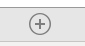button at the bottom of the screen.
2. Rename the worksheet “Summary Statistics”
• You can do this by double-clicking on the tab for your new worksheet. A dialog box will open for you to enter the new name.
3. Fill in the first column (cells A1:A9) as in the table above.

The next step is to fill in the row of variable names. We could type them in, but let’s do something more sophisticated and flexible: use a formula to pull the variable names in from the original data set.

Getting variable names from the data

To fill in the variable name for column B:

1. Go to cell B1.
2. Type “=” but don’t hit <enter> yet.
3. Click on the tab for the “Data for Analysis” worksheet, and then click on cell G1 in the Data for Analysis worksheet.
• The formula bar now says ='Data for Analysis'!G1
4. Hit <enter>.
• You should now be back in cell B1 in the Summary statistics worksheet.
• Cell B1 should display “UnempRate” (the contents of cell G1 in Data for Analysis).

What have we done here? We have constructed a formula that successfully references a cell in another worksheet. Note that the relative references are even copied over correctly.

Excel has many bulit-in functions to calculate summary statistics.

Summary statistics for UnempRate

To fill in the statistics for column B:

1. Use the COUNT() function to report the observation count in cell B2.
• We will want to use an absolute reference for the rows, and a relative reference for the columns, so the formula should be =COUNT('Data for Analysis'!G$2:G$542).
2. Use the AVERAGE() function to report the average unemployment rate in cell B3
3. Use the STDEV.S() function to report the standard deviation of the unemployment rate in cell B4
• There is another builit-in Excel function called STDEV.P() that uses a slightly different formula for the standard deviation: $s_x^p = \frac{1}{n} \sum_{i=1}^n (x_i - \bar{x})^2$ We will discuss the difference between these two statistics later.
4. Use the MIN() function to report the minimum unemployment rate in cell B5.
5. Use the PERCENTILE.INC() function to report the 10th percentile of the unemployment rate in cell B6.
• Warning: Despite its name, the PERCENTILE.INC() function takes a quantile (between 0 and 1) rather than a percentile (between 0 and 100) as its argument.
6. Use the MEDIAN() function to report the median unemployment rate in cell B7
7. Use the PERCENTILE.INC() function to report the 90th percentile of the unemployment rate in cell B8.
8. Use the MAX() function to report the maximum unemployment rate in cell B9.

We now have a table that reports all of the major summary statistics calculated for the unemployment rate.

We would also like to calculate summary statistics for other variables. Fortunately, we have set up our table in a way that makes that easy.

Summary statistics for other variables

To fill in summary statistics for other variables:

1. Copy the contents of cells B1:B9 to cells C1:F9.

Now column C contains summary statistics for the labour force participation rate (LFPRate), and columns D through F contain summary statistics for some of the other variables in our data set.

If we wanted to, we could set up the table to calculate summary statistics for all of the variables. But let’s just stop with these, and move on to making the table look a little nicer.

#### 5.2.1.2 Cleaning up the table

Our table now has all of the information we need, but it is still kind of ugly. Let’s make it look nice and presentable.

Cell size

The first problem is that the columns may be too narrow or too wide. If a column is too narrow, some of its values will display as “####”. If it is too wide, it will take up too much room.

There are many different ways to adjust row heights and column widths, but here is the simplest:

1. Select the whole sheet by clicking on the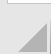button in its upper left corner.
2. Select Home > Format > AutoFit Column Width from the menu.

Not only will this make everything fit, it will automatically adjust the width as necessary when anything changes.

Error codes

The second problem is that the non-numeric variables report error codes like #DIV/0! or #NUM! for several statistics, and nonsense values for others.

It is usually better to leave something blank than have it display meaningless, confusing or incorrect information. So let’s just delete those columns:

1. Select columns D and E.
2. Select Home > Delete > Delete Sheet Columns.

Display formatting

To make the display formatting a little nicer.

1. Put the top row in bold.
2. Put the first column in italics.
3. Adjust the number display formats to look nice. Remember that the number display format has no effect on the number itself.
• Leave the counts as they are.
• Display the unemployment rate, LFP rate and population growth rate in percentages, rounded to one decimal place.
4. Feel free to play around with colors, fonts, etc. to get a table that you like.

We now have a nice table of summary statistics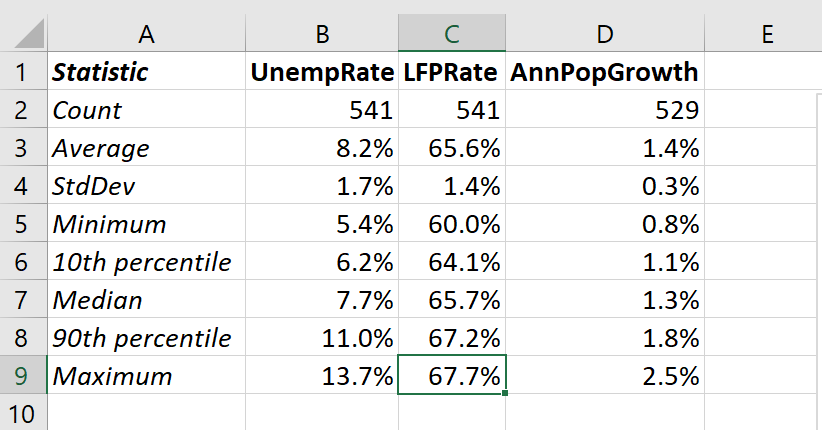Table of summary statistics

that we could put into a Word or PowerPoint document, and share with an audience.

### 5.2.2 Simple frequency tables

Another way of looking at a single variable is to construct a frequency table. A frequency table describes the distribution of values for a single variable.

Frequency tables are at their simplest and most useful when the underlying variable is discrete, categorical, or even non-numeric. The frequency table for this kind of variable looks like this:

(variablename) Count Percentage
(value1) (# value1) (% value1)
(value2) (# value2) (% value2)
(value3) (# value3) (% value3)
etc.

where:

• The (variablename) column lists all possible values of the variable.
• The Count column reports the number of observations in which the variable matches that value or range of values.
• The Percentage column reports the count as a percentage of the total number of observations.

The Excel functions COUNTIF() and COUNTIFS() can be used to construct the counts. I will use COUNTIFS() which has a pair of arguments:

• The first argument criteria_range gives the range containing the data we want to describe.
• The second argument criteria gives the criteria we want to match.

The kind of criteria you can use are most easily described by examples:

• The formula =COUNTIFS(A1:A5,"Hello") returns a count of the number of cells in the range A1:A5 that contain the string “Hello”.
• The formula =COUNTIFS(A1:A5,5) returns a count of the number of cells that contain the number 5.
• The formula =COUNTIFS(A1:A5,">0") returns a count of the number of cells in the range A1:A5 that contain a number greater than zero.
• The formula =COUNTIFS(A1:A5,B1) returns a count of the number of cells in the range A1:A5 that satisfy the criterion given in cell B1.

A simple frequency table

Let’s create a frequency table for the Party variable in our data set. It should look like this:

Party Count Percentage
Liberal (# Liberal) (% Liberal)
Conservative (# Conservative) (% Conservative)
NDP (# NDP) (% NDP)
Transfer (# Transfer) (% Transfer)

Note that I have included a political party (the NDP) that is not observed in our data set. We start by setting up the table:

1. Create a new sheet named “Party control”
2. Fill in the top row and first column as in the table above.

Next we will fill in values for the Count column (B):

1. Fill in cell B2 using the Excel function COUNTIFS().
• The criteria_range will be the data in our main data set (Data for Analysis workhseet) covering the Party variable (cell range I2:I542), or 'Data for Analysis'!I2:I542.
• The criteria we want to match is the contents of the cell in the current row of column A, or A2.
• So the formula to enter in cell B2 is =COUNTIFS('Data for Analysis'!I2:I542,A2).
• The result displayed should be 302, the number of observations in our data that have the Party identified as Liberal.
2. We will want to copy the formula in cell B2 to the other cells in column B. But before we do that, we need to make some of the relative references into absolute references. In this case, our table will keep the same criteria_range but will want to change the criteria, so:
• Change the formula in cell B2 to =COUNTIFS('Data for Analysis'!I$2:I$542,A2)
3. Copy cell B2 to cells B3:B4.
• Check the formulas in those cells to make sure they look right.

Next, we need to fill in the Percentage column (C).

1. To do that we just need to divide each cell in the Count column by the sum of all the cells in that column. So the formula in cell C2 should be =B2/SUM(B2:B5)
2. Change relative references to absolute references as needed,
3. Copy the formula in cell C2 to cells C3:C5.
4. By default, the percentages are displayed as proportions. Change the display format to percentage, with one decimal place.

We now have a simple frequency table for the Party variable.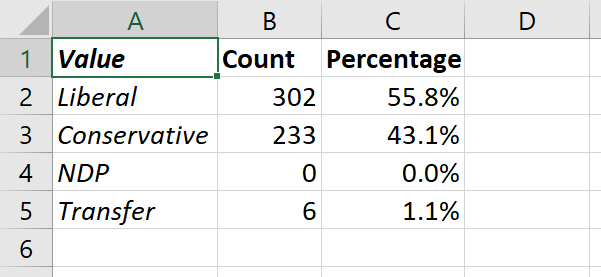Party control frequency table

### 5.2.3 Binned frequency tables

We can also construct frequency tables for continuous variables or discrete variables with many possible values, but doing so is a little more complicated. We cannot just construct a table with a row for each possible value, since there are many possible values.

Instead, we divide the data’s range of possible or observed values into a set of sub-ranges or bins. Then we can calculate and report the number or percentage of observations that fall within each bin. A binned frequency table looks like this:

From To Count Percentage
(from1) (to1)
(from2) (to2)
etc.

In constructing bins, we need to apply some good judgement, and keep in mind a few requirements and considerations:

• We need the bins to cover the full range of the data. In particular:
• The lower bound of the lowest bin should be lower than the lowest value in the data.
• The upper bound of the highest bin should be higher than the highest value in the data.
• Each bin’s upper bound should be the lower bound of the next bin.
• Boundaries should be addressed in a consistent manner, so that each observation falls into exactly one bin.
• We often want the bins to be equally sized.
• But that isn’t always the case. See the unemployment rate table in the example below; if it used equally sized bins, most of the bins would be empty.
• We often want the upper and lower bounds of the bins to be nice round numbers.
• The number of bins is a matter for judgment, and depends on what kind of patterns we are aiming to find in the data.
• Too many bins and we miss broad patterns
• Too few bins and we miss potentially interesting details.
• The solution is to explore multiple options, and see what patterns you can find out.

Again, we will use COUNTIFS() to construct the counts. But we will need to take advantage of a feature of COUNTIFS() I have not yet mentioned: it takes multiple criteria_range and criteria arguments, allowing it to make multiple comparisons (that’s the difference between COUNTIF() and COUNTIFS()).

A binned frequency table

Let’s create the following table for the unemployment rate variable:

From To Count Percentage
0.0% 5.0%
5.0% 7.5%
7.5% 10.0%
10.0% 15.0%
15.0% 100.0%

where Count is the number of observations for UnempRate that are greater than that row’s From value and less than or equal to the row’s To value, and Percentage is the count as a percentage of the total. We start by setting up the table:

1. Create a new sheet titled “Unemployment frequency”
2. Copy the first row and first two columns from the table above.
• Enter the From and To values as decimals (0 to 1) and display them as percentages (0% to 100%).

Next, we fill in the first count in cell C2. This will be a somewhat complex formula, so we will build it in stages:

1. Let’s start by counting the observations with UnempRate greater than zero:
• The criteria_range1 value should be the data range for the UnempRate variable, i.e, 'Data for Analysis'!G2:G542.
• The criteria1 value should be is the string “>0”.
• So the formula should be =COUNTIFS('Data for Analysis'!G2:G542, ">0")
2. Next, let’s add the criterion that UnempRate is less than or equal to 5%.:
• The criteria_range2 value should be the same as criteria_range1.
• The criteria2 value should be is the string “<=0.05”.
• So the formula should be =COUNTIFS('Data for Analysis'!G2:G542, ">0", 'Data for Analysis'!G2:G542, "<=0.05")
3. Finally, let’s have the formula retrieve the criteria1 and criteria2 values from cells A2 and B2.
• This enhancement is not strictly required but it has two big advantages:
1. we will be able to copy this formula into other cells
2. we can change the bins in columns A and B, and the calculation will automatically adjust.
• We can use the CONCAT() function to construct the criteria:
• CONCAT(">",A2) will return “>0”
• CONCAT("<=",B2) will return “<=0.05”
• So the formula is: =COUNTIFS('Data for Analysis'!G2:G542,CONCAT(">",A2),'Data for Analysis'!G2:G542,CONCAT("<=",B2))

Cell C2 should now display the number of months (zero) in which the Canadian unemployment rate was between 0% and 5%. To finish up the table:

1. Update the formula in cell C2 to use absolute references where appropriate.
2. Copy the contents of cell C2 to cells C3:C6.
3. Fill in the Percentage column (C) with the appropriate formula and change its display format to Percent.

We now have our binned frequency table.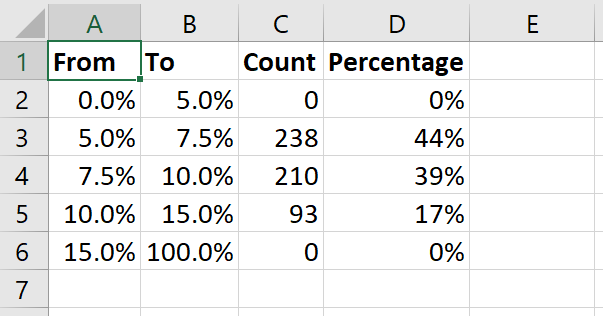Binned frequency table for UnempRate

The FREQUENCY() function is another way to ctreate a frequency table. However, this function is a tricky one to learn, and Microsoft recently changed how it works. So we will skip it.

## 5.3 Univariate graphs in Excel

Another way to explore our data is by visualizng it: constructing and viewing graphs. In this section, we will construct and view three graphs that are useful for understanding a single variable: the time series graph, the bar/column graph, and the histogram. We will also discuss some basic principles for producing presentation-quality graphs.

### 5.3.1 Charts in Excel

Excel calls graphs “charts.” Excel charts have three main components:

1. The data source. This is the table containing the data used to construct the graph.
• The individual columns in the data source are called series.
2. The chart type. There are many chart types in Excel, but the ones we will work on are:
• Line graphs have categories on the horizontal axis, and the value of a variable/series on the vertical axis. Values are depicted by a line connecting the values of the series at each category.
• Column charts have categories on the horizontal axis, and the value of a variable or series on the vertical axis. Values are depicted by a vertical bar for each value of the series.
• Bar charts are just column charts with the axes reversed.
• Scatter or XY charts plot the value of one series against the value of another series. Values can be depicted as a set of points, as a connected line, or both.
3. The chart elements, which are the different pieces of the chart
• Chart elements can be added, removed, or modified.
• The available elements vary across chart types, but can include axes, titles, legends, gridlines, and text labels.

The usual workflow here is to select the data, choose a chart type, and then modify chart elements until the chart looks the way you want it.

### 5.3.2 Time series (line) graphs

A time series graph plots one or more variables at multiple points in time. Conventionally, time is on the horizontal axis and the variable’s value is on the vertical axis. For example, we will generate a time series graph that looks like this: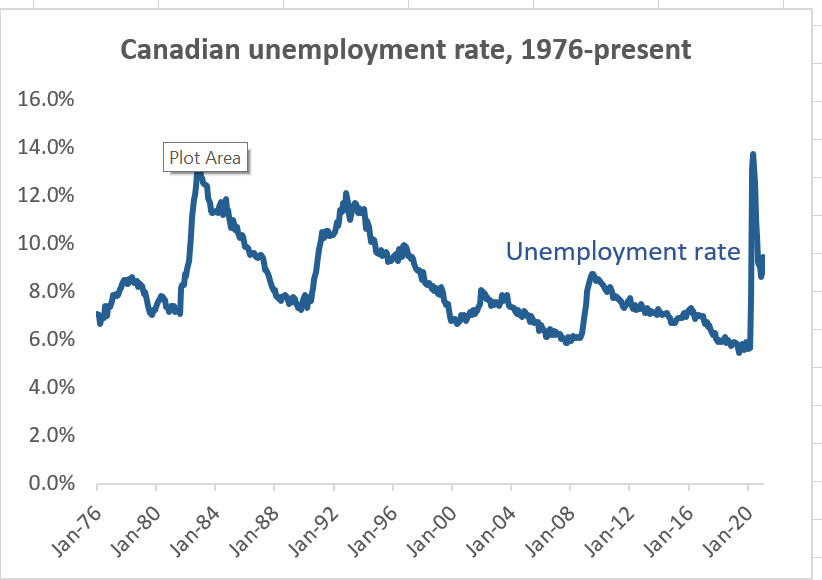Time series graph of UnempRate

In Excel, time series graphs can be implemented using either the Line chart type or the Scatter chart type. Line graphs are simpler, so we will start with that. We will learn how to make scatter plots in Chapter 11.

The first step in creating an Excel chart is to select the data source and graph type.

Creating a time series graph for UnempRate

We want to create a time series graph for our variable UnempRate.

1. Select any cell in the “Data for analysis” table.
2. Select Insert > Recommended Charts from the menu.
3. The dialog box will show a few recommended charts. None of them are what we want, so select the All Charts tab at the top to see the full set of options.
4. Select Line to see the line graph options.
5. The dialog box gives you several different types of line graph. Select the picture that looks like this: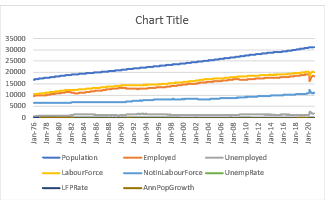and select OK.

We have our time series graph (it looks like the picture above). Unfortunately, it has a line for every variable in our data source. We only want to plot one variable, so let’s get rid of the others:

1. Select Design > Select Data which will open the Select Data Source dialog box.
2. Unckeck the checkbox next to every series in the “Legend Entries (Series)” box except UnempRate.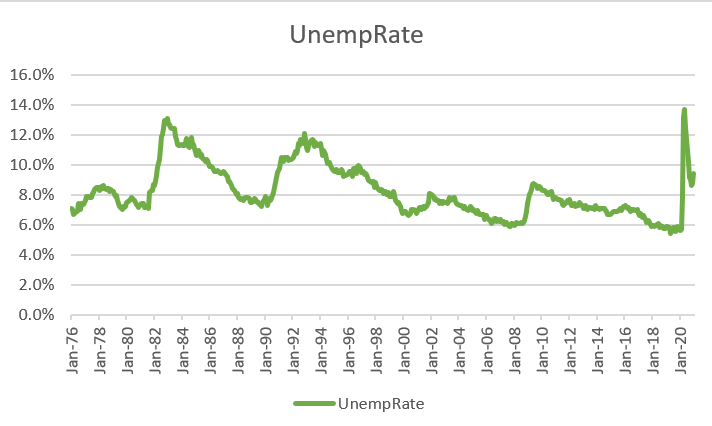Time graph series of UnempRate

This looks like the graph we want.

### 5.3.3 Creating presentation-quality graphs

The graph we have right now is perfectly fine for exploratory data analysis - using graphs and other tools to better understand the data. However, if we want to convey our understanding to others we need to fine-tune the presentation of our data. Like other elements of a presentation, graphs should be clear, informative, concise, and professional in appearance.

The field of data visualization was pioneered by the statistician Edward Tufte and studies how best to visually convey information. A full discussion of data visualization is beyond the scope of this course, but we can talk about and apply a few basic ideas:

1. The information provided should be clear.
2. All necessary information should be provided.
3. Repeated, irrelevant or misleading elements (what Tufte calls “chartjunk”) should be removed.
4. Related information should be visually “close” and required eye movement should be minimized.
5. Color and formatting should be used to simplify intepretation rather than to complicate it.
6. Accessibility should be taken into account. For example:
• Color is not always available to readers: Some readers are color blind, projectors often get colors “wrong”, or your document may get printed on a black-and-white printer.
• Always include non-color visual cues (for example dashed lines or different points with varying shape) so that readers who cannot distinguish color (for whatever reason) can tell different series apart.
• Consider using a color palette that is colorblind-friendly.
• A simple way to do this is to avoid using red and green or blue and yellow as contrasting colors, since these are the two most common forms of colorblindness.
• The geographer Cynthia Brewer has developed a set of colorblind-friendly palettes known as ColorBrewer. They are available at http://colorbrewer2.org/.
• Readers in their 50’s and above often have difficulty reading small text
• Avoid squeezing important text into a tight space.
• Generate graphics that can be rescaled.
• Readers may have more general and severe visual impairments.
• Provide alt text for all images.

Preparing our graph for presentation

Let’s start by removing unnecessary chart elements.

1. Select the chart. This will cause the Chart Design and Format menu items to apper in the menu.
2. To remove the horizontal gridlines, select Chart Design > Add Chart Element > Gridlines > Primary Major Horizontal
3. To remove the legend, select Chart Design > Add Chart Element > Legend > None

Next, let’s modify the title to be more informative. Right now, it is not clear from the graph what country’s unemployment rate this is.

1. Select the title.
2. Type in the new title, and hit the <enter> key.
• I went with the title “Canadian unemployment, 1976-present”
• I also made it boldface.

Next, let’s modify the horizontal axis to be a little less busy.

1. Double-click on the horizontal axis. The Format Axis box should appear to the right.
• It may take you more than one try to double-click on the correct object.
2. Select the button (INSERT PICTURE HERE) marked “Axis Options”.
• You can see all of the choices Excel made here based on what it sees in the data.
• Feel free to play around with these options. You can return each option to its original/default state by clicking on that option’s Reset button.
3. Change the major units to either 4 Years or 8 Years, whichever one you like better.

Finally, let’s add a text label for our time series.

1. Select the chart.
2. Select Format > Insert Text Box from the menu. The cursor will change into one that looks like this:
3. Draw the text box at the desired location on the chart and enter the text “Unemployment rate”
4. Resize and move the box to just above the line for the time series.
5. Change the color of the text to the same color as the line.

This isn’t really necessary, and violates our principle of avoiding repeated information. But it is useful when we are graphing multiple time series in the same chart, as it is more direct than a legend.

Finally, we want to add alt text for the visually impaired.

1. Select the chart.
2. Select Format > Alt Text. The Alt Text dialog box will open to the right.
3. Enter the alt text in the box.
• I used “Graph of Canadian unemployment rate from January 1976 to present.”

The graph will now look like the one above. The graph here is visually clean and simple, in part because I left out many elements that I could have included: axis labels (not needed because the units are obvious from context), a fancy background, etc.

One consideration that often comes up when constructing graphs is whether the vertical axis should start at zero when the range of data does not reach zero. In our time series graph of the unemployment rate, Excel included 0% by default even though the variable never went below 5%. I could have changed the vertical axis to start at 5%, but decided not to.

There is no strict rule for whether to include zero, but we can consider the general principle described earlier: our graph should be informative and not misleading.

For further reading

Data visualization skills are valuable in the academic world and in the professional world. If you are interested in developing your skills further, you might consider our course ECON 334: Data Visualization and Economic Analysis.

You might also get a book on data visualization, either Kieran Healy’s Data Vizualization or Cole Nussbaumer Knaflic’s Storytelling with Data. Healy’s book is aimed at a primarily academic audience while Knaflic’s is aimed at a business audience. Both of them are practical and easy reads, with many examples.

### 5.3.4 Frequency (bar/column) graphs

Bar graphs are used to represent the frequency distribution of a categorical variable. Bar graphs use bars of different length to represent the value of some aggregate variable in each category.

Bar graphs are produced from a frequency table, as they are a visual depiction of the information in such a table.

They can be produced in Excel using either the Bar chart type or the Column chart type. The difference between the two is that the bars are horizontal in the Bar chart type and vertical in the Column chart type.

A bar graph of the Party variable

To construct a bar graph of the Party variable:

1. Select any cell in the “Party control” worksheet.
2. Select Insert > Recommended Charts from the menu.
3. Select the “All Charts” tab to see the full list of options.
4. Select “Column” and then select OK.

The graph will look something like this: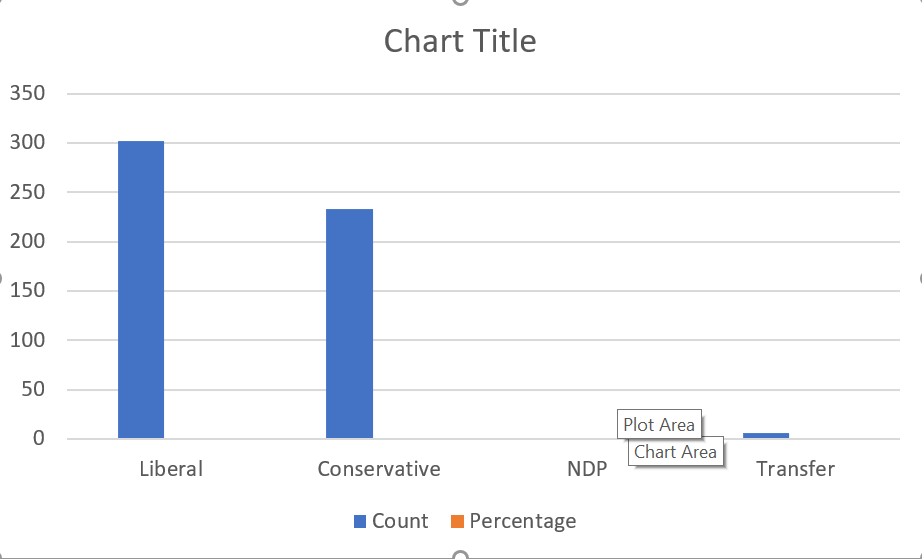Bar graph of Party variable, default options

As you can see, basic bar graphs are quite simple. There is a bar for each category (the first column of the table) and variable (the other columns), and the length of each bar corresponds to its value.

As with our line graph, the current graph contains more information than we actually want - it shows the count and the percentage, and we really only need one of those.

1. Select the chart.
2. Select Chart Design > Select Data
3. Uncheck the check boxes for Percentage, NDP and Transfer.

We now have a bar graph that looks like this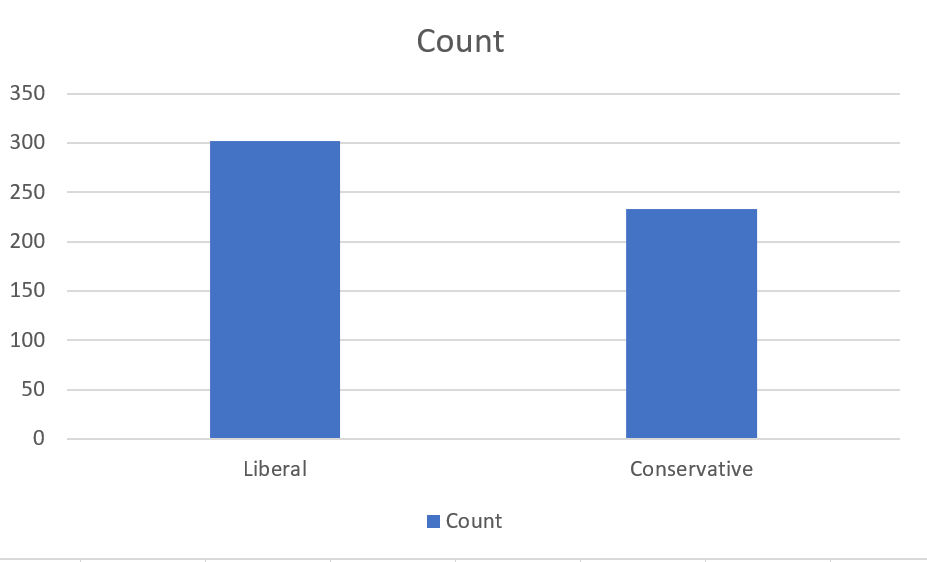Bar graph of Party variable, simplified

and shows the number of months in which the two largest federal parties were in government over the time frame of our data.

As with line graphs, we can prepare a bar graph for presentation by using Excel’s tools with an eye towards the principles of effective presentation graphics.

Cleaning up our bar graph

We can do a few easy things to simplify and clarify our bar graph:

1. Change the title.
2. Remove the gridlines.
3. Remove the legend.
4. Add the vertical axis title “Months in control” to clarify the units.

Another thing we can do is use color and branding to convey information: each major Canadian political party has a distinctive color as part of its brand: red for the Liberals, blue for the Conservatives, and orange for the NDP.

1. Double-click on the “Liberal” bar.
2. Change its color to red.
3. Double-click on the “Conservative” bar
4. Change its color to blue. The conservatives use two shades of blue, so pick whichever one you like best.

Finally, the purpose of a bar graph is to enable the viewer to compare magnitudes, which requires looking at the top of each bar. But you may notice that the category labels are at the bottom of each bar. Following the principle that we don’t want to make the reader’s eye do any extra work, let’s put those labels on top.

1. Select the chart.
2. Select Chart Design > Add Chart Element > Data Labels > More data label options.
You will notice the numbers 302 and 233 (the heights of the two bars) appear above the bars, and the Format Data Labels box will appear to the right.
3. Select Label options from the dialog box.
4. Uncheck “Value” and check “Category Name”. The category names “Liberal” and “Conservative” will now appear above the respective bar.
5. Select Chart Design > Add Chart Element > Axes > Primary Horizontal to eliminate the category names from the bottom of each bar.
6. You might also consider changing the color of the two labels to match the color of the corresponding bar.

Our final graph looks like this: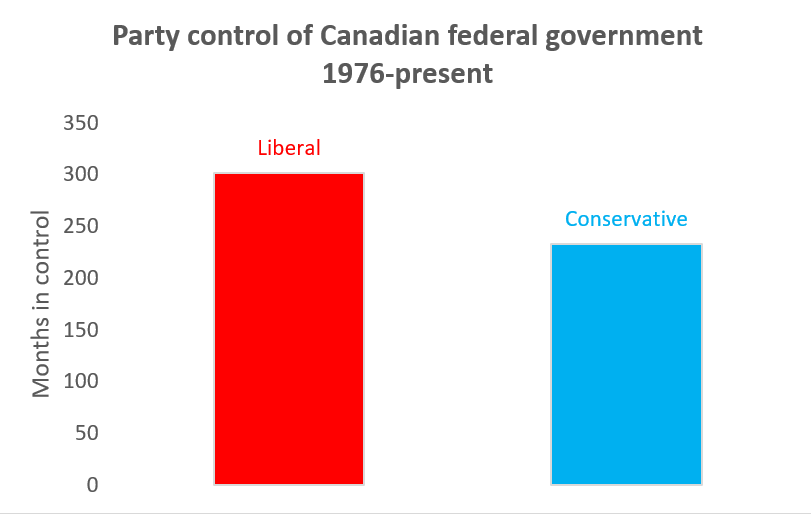Bar graph of Party variable, final version

One of the keys to effective bar graph design is to keep the graph simple and clean, and to avoid “chartjunk.”

First, bar graphs should always start at zero. The size of the bar is meant to visually represent the value of the variable it is depicting. Using any origin other than zero can cause relative sizes to be misleading.

Why bar graphs should always start at zero

Suppose we start our Party bar graph at 200 rather than zero. We will get this: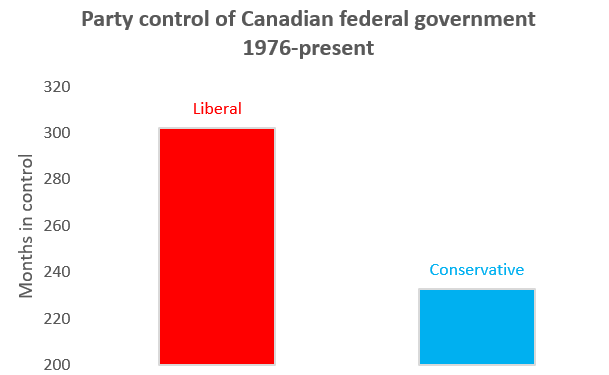Bar graph for Party, starting at 200

The relative size of these bars is very misleading. The bar for Liberal is three times as big as the bar for Conservative, even though the number it represents is only 29% higher.

Excel provides several popular alternatives to bar graphs. Pie graphs are another way of depicting relative frequencies: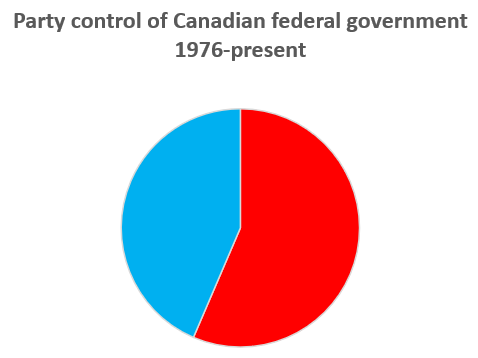Pie chart of Party variable

However, most data visualization experts recommend against their use. Research on how people process visual information usually find that they are less informative in practice. People are much better at evaluating the relative size of two lines or rectangles than they are at evaluating the relative size of two pie slices.

Similar considerations apply to 3D pie charts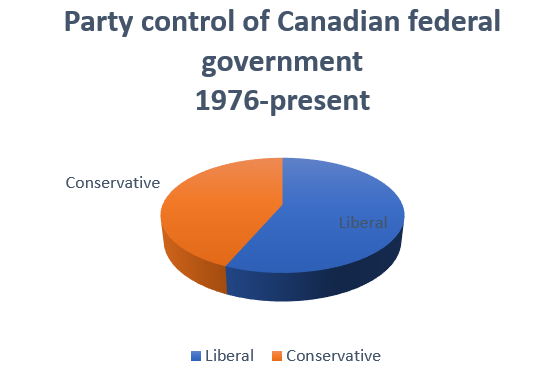and 3D bar charts.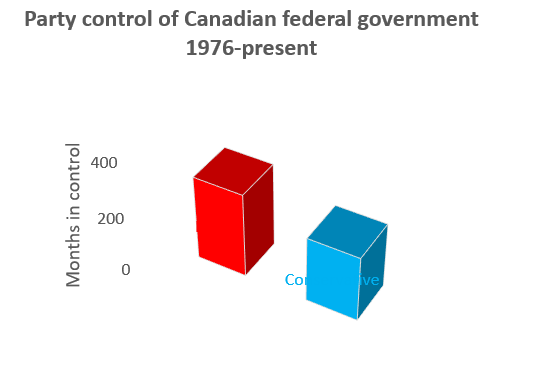Again, research indicates people are much better at assessing relative distances in two dimensions than they are in three dimensions.

### 5.3.5 Histograms

A histogram is just a frequency bar/column graph for a continuous variable. It is constructed by sorting the variable’s values into some number of equally-sized bins and then plotting a bar for each bin that represents counts or percentages.

The histogram is a built-in chart type in Excel.

Constructing a histogram for UnempRate

To construct a histogram of the UnempRate variable:

1. Select column G in the “Data for Analysis” worksheet.
• Notice that for the other charts we could just select any cell in this worksheet. For some reason, this won’t work with the histogram chart type.
2. Select Insert > Recommended Charts" from the menu bar.
3. Slect the All Charts tab to see the full set of options.
4. Select Histogram and then OK.

Our graph will appear: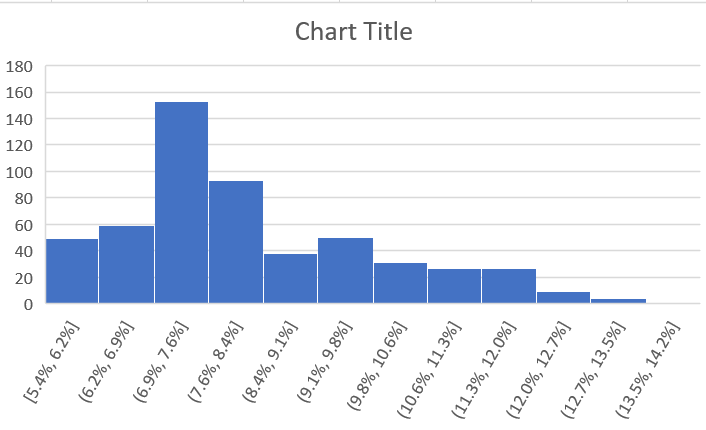Histogram of UnempRate, with default options

There are two main choices in histogram design:

• How many bins and how should they be determined?
• Should we depict frequencies as counts or percentages?

Excel allows us to customize the bins in various ways. Unfortunately, Excel histograms depict frequencies as counts, and do not easily allow for them to be displayed as percentages. We will use R to generate those histograms.

Changing the bins for a histogram

By default, Excel has chosen 12 equally-sized bins, and each covers 0.73 percentage points. This is a reasonable starting point, but we may find another choice will produce a more informative histogram.

First, let’s try varying the number of bins.

1. Select or double-click on the horizontal axis to open the “Format Axis” box.
2. Select Axis Options.
3. Select Number of bins and change the number from 12 to something else.
• Try a large number like 100 to see what a lot of small bins looks like.
• Try a small number like 5 to see what a few large bins look like.

Looking at these graphs, a relatively large number of bins is needed to accurately convey the distribution of the unemployment rate. But using a lot of bins makes the horizontal axis very busy and difficult to read. One alternative that will help is to fix the bin size at some nice round number like 1% (0.01) or 0.5% (0.005).

1. Select or double-click on the horizontal axis to open the “Format Axis” box.
2. Select Axis Options.
3. Select Bin width` and change the number to 0.01.

Now the bins are exactly one percentage point wide, which makes the horizontal axis a little easier to read and interpret.

It would also be nice to modify the start and end points so that instead of the bins being 5.4-6.4%, 6.4-7.4%, etc. they were 5-6%, 6-7%, etc. Unfortunately, that does not seem to be possible in Excel. We can work around that limitation by creating the frequency table, and making a column/bar chart of that frequency table.

Finally, as with other graphs we will want to add, delete and modify various elements to bring this histogram closer to presentation quality.

Enhancing the quality of our histogram

To enhance the quality of this histogram:

1. Add/modify the title to “Histogram of the Canadian unemployment rate, 1976-present”
2. Add the horizontal axis title “Unemployment rate”
3. Add the vertical axis title “# of months”

Our final histogram will look like this: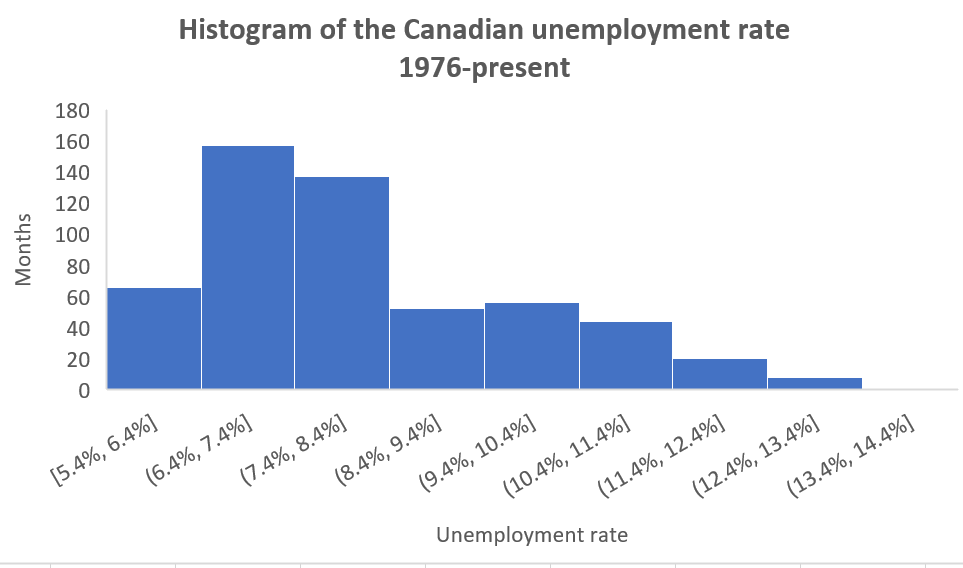Histogram of UnempRate, final version

You can download the complete Excel file with all analysis from this chapter at https://bvkrauth.github.io/files/CanadaEmploymentHistoricalWithAnalysis.xlsx.